#FreeStyler Wiki

### Site Tools

fixture_control:fanning_panel

# Panel Description - Fanning

Fanning is as an oriental ribbon fan spreading out the beams for more fixtures.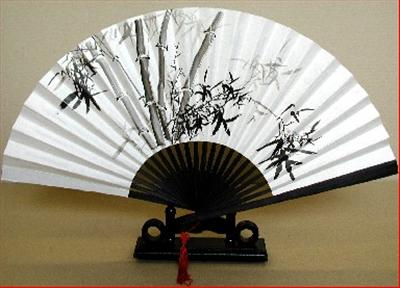This means that the buttons in this part of the movement panel only have effect when more fixtures are selected and not on one alone.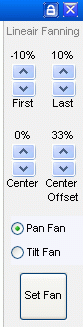When more fixtures are placed next to each other the beams may be one big spot because all beams are parallel.
By using fanning it is possible to spread out the beams as shown bellow.

Parallel beams.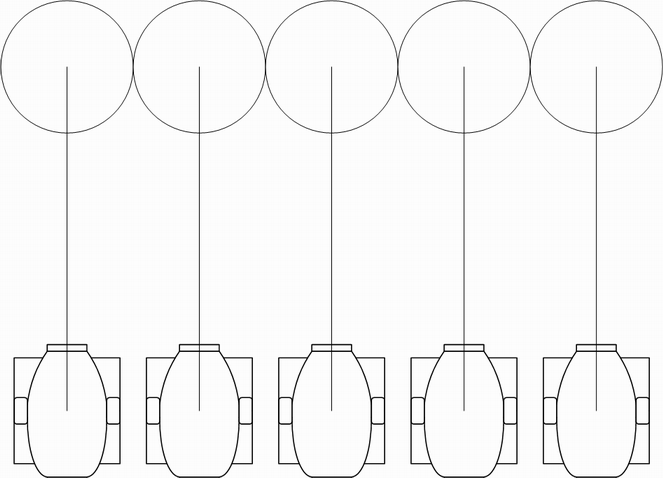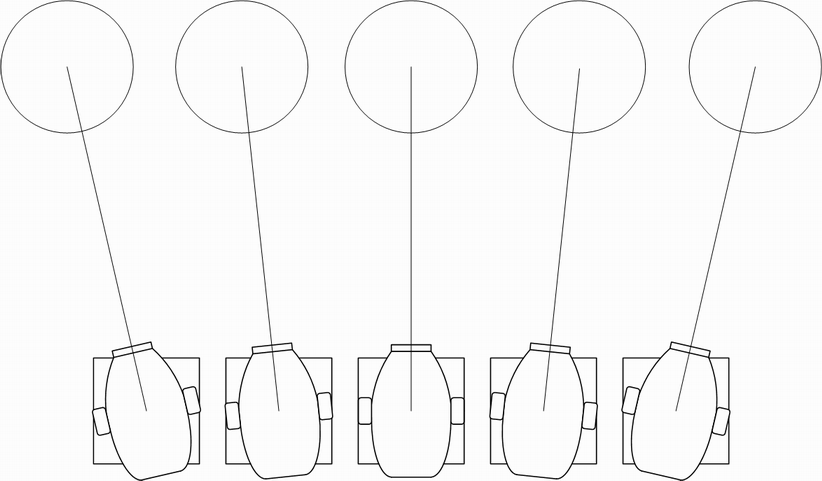Here the settings is “First” = -10% (First is defined as left Fixture), “Last” = 10% (Last is defined as right fixture), “Pan Fan” or “Set Fan”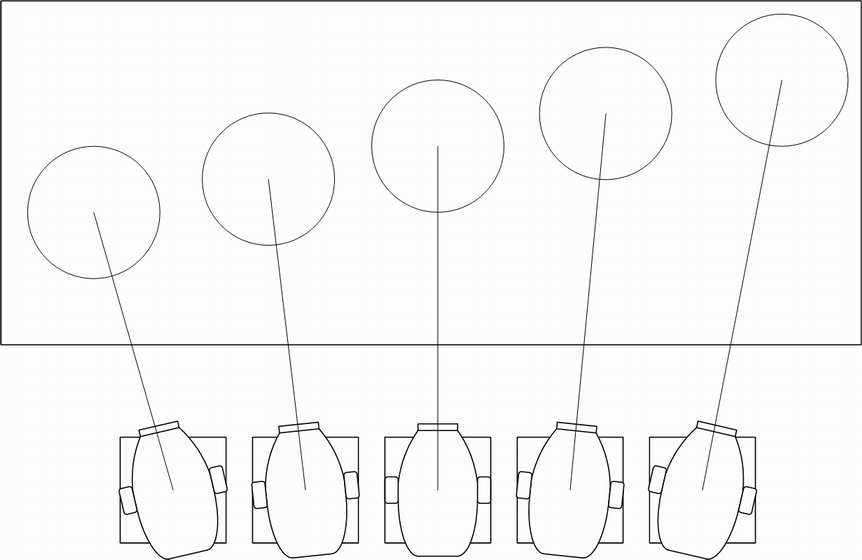The same can be done with the Tilt.

Additional center of the fan can be moved with the “Center” slider.
By adjusting the center of the fan then the spread is different from one side to an other.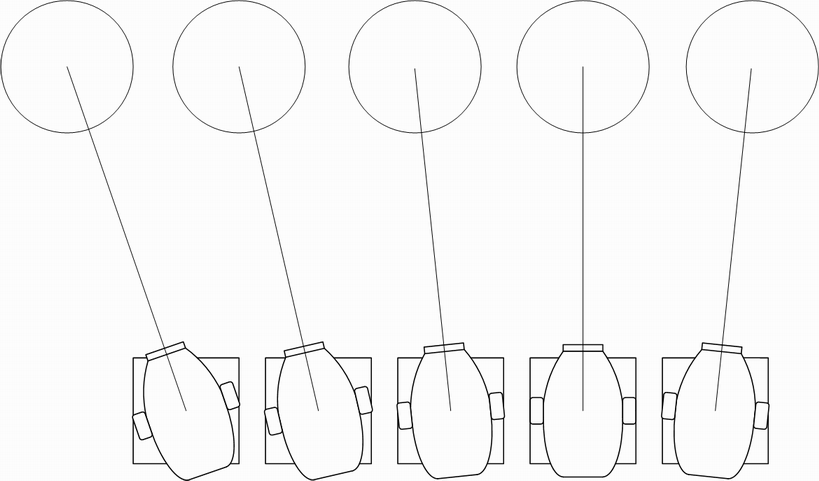Here the settings are “First” = -15%, “Last” = 5% and “Center” = -5%.
The spread will then be -15, -10, -5, 0 and 5%

Center Offset.
The parameters above is related to the beams pointing direction and in the figures the fixture is placed with the same spacing.
If the fixture is placed with unequal distance then “Center Offset” are to be used.
Center “Offset” may be a inproper word because when the Center fixture is exactly in the middle the offset is 50% meaning that it is actually describing the Center fixture placement.
Bellow are two example with Center offset. The optimal use are to be tested in practice.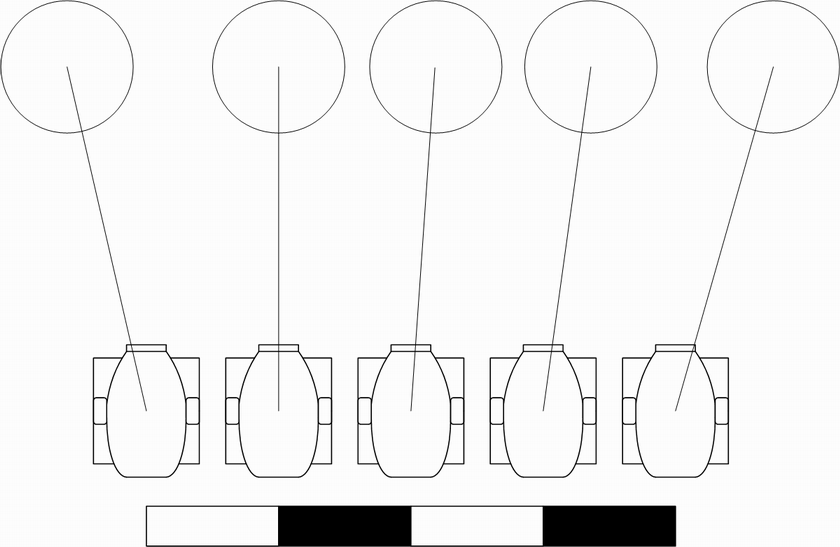In this figure the “Center Offset” is 25% meaning that fixture no. 2 from left is center.

The left fixture is 0 and the right fixture is 100%
In this figure the distance between the fixtures is 1,1,2,1. The total distance is therefore 4.
So the fixture is placed in the following distance.
Fixture 1: 0%
Fixture 2, Center: (100/4)*1 = 25%
Fixture 3: (100/4)*(1+1) = 50%
Fixture 4: (100/5)*(1+1+1) = 75%
Fixture 5: 100%

And the First = -10%, Last = 10% and Center = 0%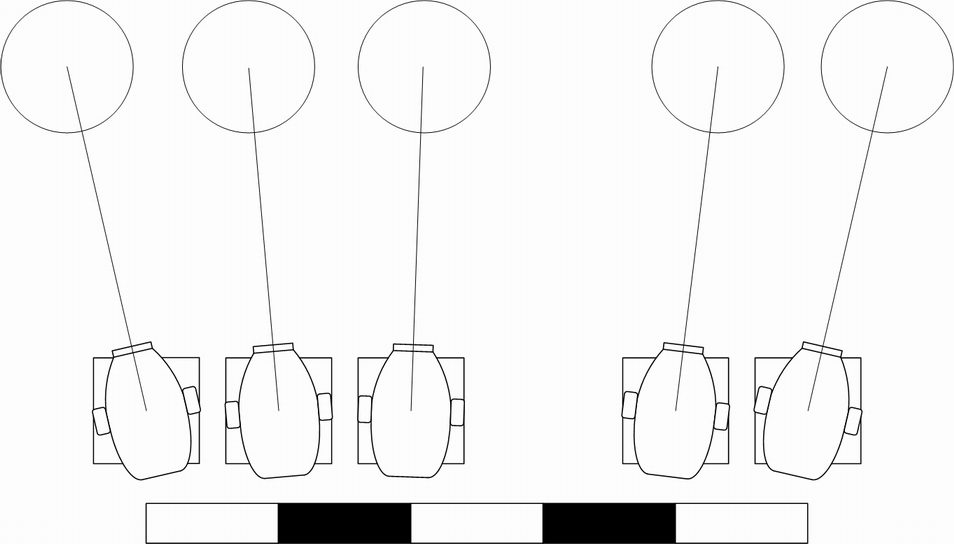In this figure the “Center Offset” is 40%. Here fixture no. 3 from left is the center but there is a spacing between the center fixture and the next.

The left fixture is 0 and the right fixture is 100%
In this figure the distance between the fixtures is 1,1,2,1. The total distance is therefore 5.
So the fixture is placed in the following distance.
Fixture 1: 0%
Fixture 2: (100/5)*1 = 20%
Fixture 3, center: (100/5)*(1+1) = 40%
Fixture 4: (100/5)*(1+1+2) = 80%
Fixture 5: 100%

And the First = -10%, Last = 10% and Center = 0%

Note:
To get the optimum performance it is important to set this offset else the beams will not track when they are moved to the outer values.